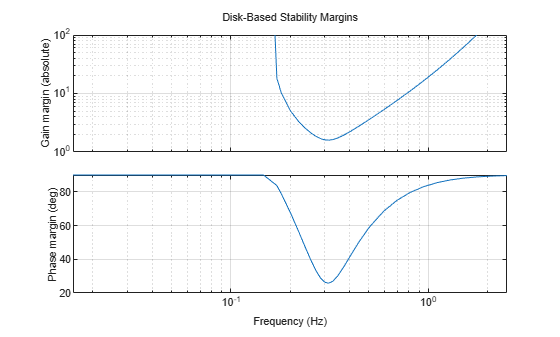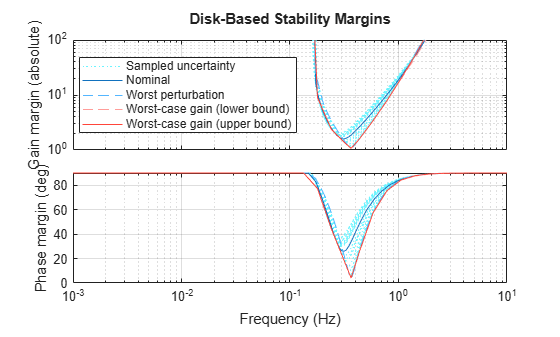# diskmarginoptions

Customize disk-based stability-margin plots

## Syntax

``opts = diskmarginoptions``
``opts = diskmarginoptions('cstprefs')``

## Description

example

``` `opts = diskmarginoptions` returns the default option set for plots you generate with `diskmarginplot` and `wcdiskmarginplot`. You can then use dot notation to change option values. Use `opts` to customize the plot appearance. ```

example

````opts = diskmarginoptions('cstprefs')` initializes the plot options with the options you have specified in the Control System Toolbox™ Preferences Editor. For more information about the editor, see Toolbox Preferences Editor. ```

## Examples

collapse all

Plot the disk margins as a function of frequency of a system with the following open-loop response.

`L = tf(25,[1 10 10 10]);`

For the plot, specify the following attributes:

• Frequency units: Hz

• Gain margins on a log scale, in absolute units

• Grid on

```opts = diskmarginoptions; opts.FreqUnits = 'Hz'; opts.MagScale = 'log'; opts.MagUnits = 'abs'; opts.grid = 'on'; diskmarginplot(L,opts)```Plot the worst-case disk margins as a function of frequency of a system with the following open-loop response.

```a = ureal('a',10,'PlusMinus',[-4,4]); L = tf(25,[1 a a a]);```

For the plot, use the default preferences specified in your Control System Toolbox preference, except specify the following attributes:

• Frequency units: Hz

• Gain margins on a log scale, in absolute units

• Grid on

```opts = diskmarginoptions('cstprefs'); opts.FreqUnits = 'Hz'; opts.MagScale = 'log'; opts.MagUnits = 'abs'; opts.grid = 'on'; w = {2*pi*1e-3,2*pi*10}; % rad/s wcdiskmarginplot(L,w,opts)```The plot you obtain might differ in appearance, depending on your current Control System Toolbox preference settings. (See Toolbox Preferences Editor.)

## Output Arguments

collapse all

Options for disk-margin plots of dynamic systems, returned as a `diskmarginplot` options set. Initialize the value of `opts` with either default options or the values specified in the Control System Toolbox preferences editor. Customize the option values using dot notation. Then, use `opts` with `diskmarginplot` or `wcdiskmarginplot` to customize plots of the disk-based stability margins of dynamic systems. The following table lists the available options.

Option Description
`FreqUnits`

Frequency units, specified as one of the following:

• `'rad/second'` (default)

• `'auto'` — Use frequency units `rad/TimeUnit` relative to system time units specified in the `TimeUnit` property of the dynamic system.

• `'Hz'`

• `'rpm'`

• `'kHz'`

• `'MHz'`

• `'GHz'`

• `'rad/nanosecond'`

• `'rad/microsecond'`

• `'rad/millisecond'`

• `'rad/minute'`

• `'rad/hour'`

• `'rad/day'`

• `'rad/week'`

• `'rad/month'`

• `'rad/year'`

• `'cycles/nanosecond'`

• `'cycles/microsecond'`

• `'cycles/millisecond'`

• `'cycles/hour'`

• `'cycles/day'`

• `'cycles/week'`

• `'cycles/month'`

• `'cycles/year'`

`FreqScale`

Frequency scale, specified as:

• `'log'` (default)

• `'linear'`

`MagUnits`

Units of gain margin, specified as:

• `'dB'` (default)

• `'abs'` — Absolute units

`MagScale`

Scale of gain-margin plot, specified as:

• `'linear'` (default)

• `'log'`

`PhaseUnits`

Units of phase margin, specified as:

• `'deg'` (default)

• `'rad'`

`Title`, `XLabel`, `YLabel`

Title and axis label text and style, specified as a structure with the following fields:

• `String` — Label of the title, x-axis, or y-axis

• `FontSize`

• `FontWeight``'Normal'` (default) or `'Bold'`

• `FontAngle``'Normal'` (default) or `'Italic'`

• `'Color'``[0 0 0]` (default)

• `Interpreter``'tex'` (default)

`TickLabel`

Tick label style, specified as a structure with the following fields:

• `FontSize`

• `FontWeight``'Normal'` (default) or `'Bold'`

• `FontAngle``'Normal'` (default) or `'Italic'`

• `'Color'``[0 0 0]` (default)

`Grid`

Show or hide the grid, specified as:

• `'off'` (default)

• `'on'`

`GridColor`

Color of grid lines, specified as a vector of RGB values in the range `[0,1]`. The default value is `[0.15,0.15,0.15]`.

`XlimMode`, `YlimMode`

Selection mode for axis limits, specified as:

• `'auto'` (default) — Set axis limits automatically based on plotted data.

• `'manual'` — Specify axis limits using `XLim` and `YLim` options.

`Xlim`, `Ylim`

Axis limits, specified as a two-element vector of the form `[min,max]`.

## Version History

Introduced in R2020a